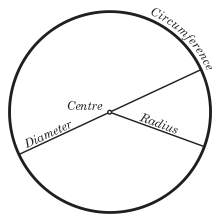Circle illustration

In classical geometry, a radius of a circle or sphere is any line segment from its center to its perimeter. By extension, the radius of a circle or sphere is the length of any such segment, which is half the diameter. If the object does not have an obvious center, the term may refer to its circumradius, the radius of its circumscribed circle or circumscribed sphere. In either case, the radius may be more than half the diameter, which is usually defined as the maximum distance between any two points of the figure. The inradius of a geometric figure is usually the radius of the largest circle or sphere contained in it. The inner radius of a ring, tube or other hollow object is the radius of its cavity.

For regular polygons, the radius is the same as its circumradius. The inradius of a regular polygon is also called apothem. In graph theory, the radius of a graph is the minimum over all vertices u of the maximum distance from u to any other vertex of the graph.

The name comes from Latin radius, meaning "ray" but also the spoke of a chariot wheel.  The plural of radius is radii.

## Formulas for circles

The radius of the circlest with perimeter (circumference) C is$r = \frac{C}{2\pi}= \frac{C}{\tau}.$

The radius of a circle with area A is$r= \sqrt{\frac{A}{\pi}}.$

To compute the radius of a circle going through three points P1, P2, P3, the following formula can be used:$r=\frac{|P_1-P_3|}{2\sin\theta}$

where θ is the angle$\angle P_1 P_2 P_3.$

This formula uses the Sine Rule.

## Formulas for regular polygons

These formulas assume a regular polygon with n sides.

The radius can be computed from the side s by:$r = R_n\, s$    where$R_n = \frac{1}{2 \sin \frac{\pi}{n}} \quad\quad \begin{array}{r|ccr|c} n & R_n & & n & R_n\\ \hline 2 & 0.50000000 & & 10 & 1.6180340- \\ 3 & 0.5773503- & & 11 & 1.7747328- \\ 4 & 0.7071068- & & 12 & 1.9318517- \\ 5 & 0.8506508+ & & 13 & 2.0892907+ \\ 6 & 1.00000000 & & 14 & 2.2469796+ \\ 7 & 1.1523824+ & & 15 & 2.4048672- \\ 8 & 1.3065630- & & 16 & 2.5629154+ \\ 9 & 1.4619022+ & & 17 & 2.7210956- \end{array}$

## Formulas for hypercubes

The radius of a d-dimensional hypercube with side s is$r = \frac{s}{2}\sqrt{d}.$

Wikimedia Foundation. 2010.

### Look at other dictionaries:

• RADIUS — (Akronym für Remote Authentication Dial In User Service, Authentifizierungsdienst für sich einwählende Benutzer) ist ein Client Server Protokoll, das zur Authentifizierung, Autorisierung und zum Accounting (Triple A System) von Benutzern bei… …   Deutsch Wikipedia

• radius — [ radjys ] n. m. • 1538; mot lat. « rayon » ♦ Os long, situé à la partie externe de l avant bras, en dehors du cubitus (⇒ radial). ● radius nom masculin (latin radius, rayon) Le plus externe des deux os de l avant bras, articulé avec le cubitus.… …   Encyclopédie Universelle

• RADIUS — Saltar a navegación, búsqueda RADIUS (acrónimo en inglés de Remote Authentication Dial In User Server). Es un protocolo de autenticación y autorización para aplicaciones de acceso a la red o movilidad IP. Utiliza el puerto 1813 UDP para… …   Wikipedia Español

• radius — RÁDIUS s.n. Os lung care, împreună cu cubitusul, formează scheletul antebraţului. [pr.: di us] – Din fr., lat. radius. Trimis de RACAI, 22.11.2003. Sursa: DEX 98 ﻿ rádius s. n. (sil. di us) Trimis de siveco, 10.08.2004. Sursa: Dicţionar… …   Dicționar Român

• Radius — Ra di*us (r[=a] d[i^]*[u^]s), n.; pl. L. {Radii} (r[=a] d[i^]*[imac]); E. {Radiuses} (r[=a] d[i^]*[u^]s*[e^]z). [L., a staff, rod, spoke of a wheel, radius, ray. See {Ray} a divergent line.] 1. (Geom.) A right line drawn or extending from the… …   The Collaborative International Dictionary of English

• RADIUS — Cette page d’homonymie répertorie les différents sujets et articles partageant un même nom. Radius peut s utiliser dans différents sens : Radius, un os de l avant bras, nervure radiale, selon le système Comstock Needham de nommage des… …   Wikipédia en Français

• RADIUS — apud Virgilium, de Conone et Archimede, Ecl. 3. v. 41. In medio duo signa, Conon: et quis fuit alter, Descripsit totum radio qui gentibus orbem: Servio, est virga Philosophorum, quâ Geometra lineas indicant. Inventa autem haec Ars est tempore,… …   Hofmann J. Lexicon universale

• RADIUS — (acrónimo en inglés de Remote Access Dial In User Server). Es un protocolo de autentificación, autorización y accounting para aplicaciones de acceso a la red o movilidad IP. Cuando se realiza la conexión con un ISP mediante …   Enciclopedia Universal